# Square pyramid

Square pyramid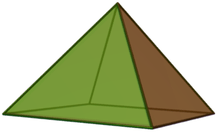Type Johnson
J92J1J2
Faces 4 triangles
1 square
Edges 8
Vertices 5
Vertex configuration 4(32.4)
(34)
Schläfli symbol ( ) ∨ {4}
Symmetry group C4v, , (*44)
Rotation group C4, +, (44)
Dual polyhedron self
Properties convex
Net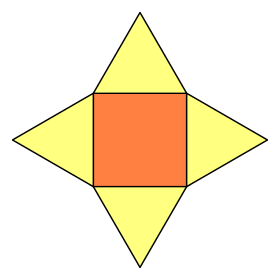In geometry, a square pyramid is a pyramid having a square base. If the apex is perpendicularly above the center of the square, it will have C4v symmetry.

## Johnson solid (J1)

If the sides are all equilateral triangles, the pyramid is one of the Johnson solids (J1). The 92 Johnson solids were named and described by Norman Johnson in 1966.

A Johnson solid is one of 92 strictly convex polyhedra that have regular faces but are not uniform (that is, they are not Platonic solids, Archimedean solids, prisms or antiprisms). They were named by Norman Johnson, who first listed these polyhedra in 1966.

The Johnson square pyramid can be characterized by a single edge-length parameter a. The height H (from the midpoint of the square to the apex), the surface area A (including all five faces), and the volume V of such a pyramid are:## Other square pyramids

Other square pyramids have isosceles triangle sides.

For square pyramids in general, with base length l and height h, the surface area and volume are: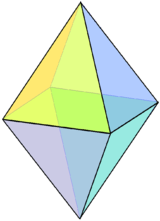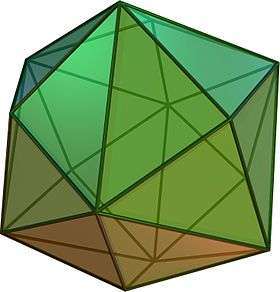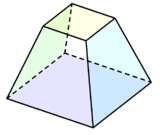A regular octahedron can be considered a square bipyramid, i.e. two Johnson square pyramids connected base-to-base. The tetrakis hexahedron can be constructed from a cube with short square pyramids added to each face. Square frustum is a square pyramid with the apex truncated.

Square pyramid fill the space with tetrahedron or truncated cube or cuboctahedron.

### Dual polyhedron

The square pyramid is topologically a self-dual polyhedron. The dual edge lengths are different due to the polar reciprocation.

Dual Square pyramid Net of dual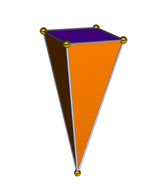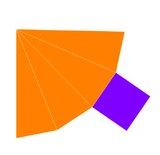## Topology

Like all pyramids, the square pyramid is self-dual, having the same number of vertices as faces.

A square pyramid can be represented by the Wheel graph W5.